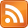# kaashif's blog

Programming, with some mathematics on the side## Quaternions, spinors and rotations

### 2014-12-26

Earlier, I was trying to find something I could talk about at my school's maths society. It had to be something exciting, useful, or at least beautiful in some way. I really wanted to do something on quaternions and vectors, because it seemed fun. The problem came when I realised I had to do something more substantial than stand there and explain something that boring. Then I saw this quote:

No one fully understands spinors. Their algebra is formally understood but their general significance is mysterious. In some sense they describe the "square root" of geometry and, just as understanding the square root of -1 took centuries, the same might be true of spinors. Michael Atiyah

Wow, that sounds cool. Maybe I'll be the one to explain spinors to everyone.

## Some basics

Before delving into the nitty gritty of what a spinor actually is, there are a few things that need to be understood. This is a matrix:

$$M = \begin{bmatrix} a & b \ c & d \end{bmatrix}$$

This is that same matrix, transposed:

$$MT = \begin{bmatrix} a & c \ b & d \end{bmatrix}$$

This is the conjugate transpose, usually denoted by an asterisk or dagger:

$$M^{\dagger} = \begin{bmatrix} a^\ast & c^\ast \ b^\ast & d^\ast \end{bmatrix}$$

This matrix $M$ is a unitary matrix, if and only if:

• $M^{\dagger}M = MM^{\dagger} = I$
• Multiplying by $M$ preserves the inner product of two complex vectors. i.e. $Mx \cdot My = x \cdot y$
• $|\det(M)| = 1$

In case you're wondering, the inner product of two vectors in $\mathbb{C}^2$ is defined as:

$$(a,b) \cdot (c,d) = ac^\ast + bd^\ast$$

This is known as the Hermitian inner product.

There are a few other conditions for a matrix to be unitary, but they'd require a longer explanation. In any case, the most important parts are there.

Importantly, all of these properties mean that unitary $n \times n$ matrices form a group, $U(n)$ under matrix multiplication.

If we take all of the elements of $U(n)$ with determinant 1, we get a special unitary group, $SU(n)$.

## Special cases of special groups

There are loads of things you can do with these groups (mostly to do with quantum mechanics), but something that really caught my eye was the fact that there exists an injective mapping from $SU(2)$ to $\mathbb{H}$. That is interesting. Obviously, the mapping can't be a bijection, since not all quaternions correspond to unitary matrices. Maybe that's not obvious yet.

To see how $\mathbb{H}$ relates to $2 \times 2$ matrices, let's look at the mapping from from $\mathbb{C}$. Complex numbers in the form $a+bi$ can be represented by a real matrix in the form:

$$\begin{bmatrix} a & -b \ b & a \end{bmatrix}$$

Using that, it seems natural to say that $\mathbb{H}$ is isomorphic to $2 \times 2$ complex matrices in the form:

$$X = \begin{bmatrix} \alpha & \beta \ -\beta^\ast & \alpha^\ast \end{bmatrix}$$

Where $X = a + b\mathbf{i} + c\mathbf{j} + d\mathbf{k}$, $\alpha = a+bi$ and $\beta = c + di$.

If we remember the handy quaternion multiplication table, use the right hand rule for vector multiplication, or use this image (very intuitive), we remember that:

$$\mathbf{i}\mathbf{j} = \mathbf{k},\;\; \mathbf{j}\mathbf{k} = \mathbf{i},\;\; \mathbf{k}\mathbf{i} = \mathbf{j}$$

The only way you can get a matrix in the form above and stick to these rules is to define the unit quaternions as:

$$\mathbf{i} = \begin{bmatrix} i & 0 \ 0 & -i \end{bmatrix}!,\;\; \mathbf{j} = \begin{bmatrix} 0 & 1 \ -1 & 0 \end{bmatrix}!,\;\; \mathbf{k} = \begin{bmatrix} 0 & i \ i & 0 \end{bmatrix}$$

Obviously, the only quaternions that will end up with determinant 1 will be the ones of norm 1 - the unit quaternions. Thus, the mapping is obviously injective, since not all quaternions have this.

The norm of a quaternion is simple to work out, since (intuitively) norm is a generalised idea of the length of a vector. For example, for the quaternion $X$ I mentioned earlier, its norm would be $\sqrt{a2+b2+c2+d2}$.

Just a note: if you want to multiply quaternions $X$ and $Y$, it's easier to treat their real parts and imaginary parts separately. If we say $X = (x,\vec{x})$ and $Y=(y,\vec{y})$:

$$XY = (xy - \vec{x} \cdot \vec{y}) + (x\vec{y} + y\vec{x} + \vec{x} \times \vec{y})$$

This is a lot easier because $\vec{x}$ and $\vec{y}$ are just ordinary vectors in $\mathbb{R}^3$, which are very easy to deal with.

## What use is this?

That seems really useless, but remember that quaternions are widely used in computer graphics (among other places) to represent rotations in three dimensions, which means $SU(2)$ is also isomorphic to $SO(3)$. Not only that, there are dozens of uses in quantum mechanics to do with spin, QCD and, you guessed it, spinors.

But what is a spinor? You could find out on the Wikipedia page, but chances are you won't understand any of it without either spending a long time trawling through a massive tree of articles or having someone explain it.

I would explain it now, but I have other things to do.

## That's it?! But I still have no idea what a spinor is!

Well then, I guess you should come to Maths Society on Thursday the 5th of February!

While I definitely won't have anything finished before the day, you can check out any work in progress at http://kaashif.co.uk/code/spinor.git/.